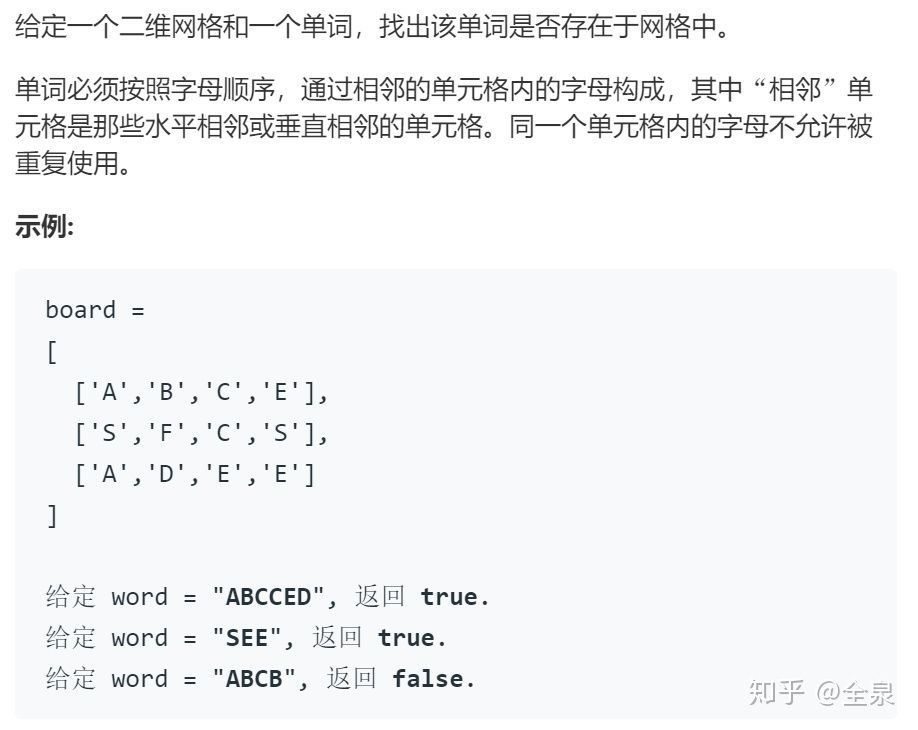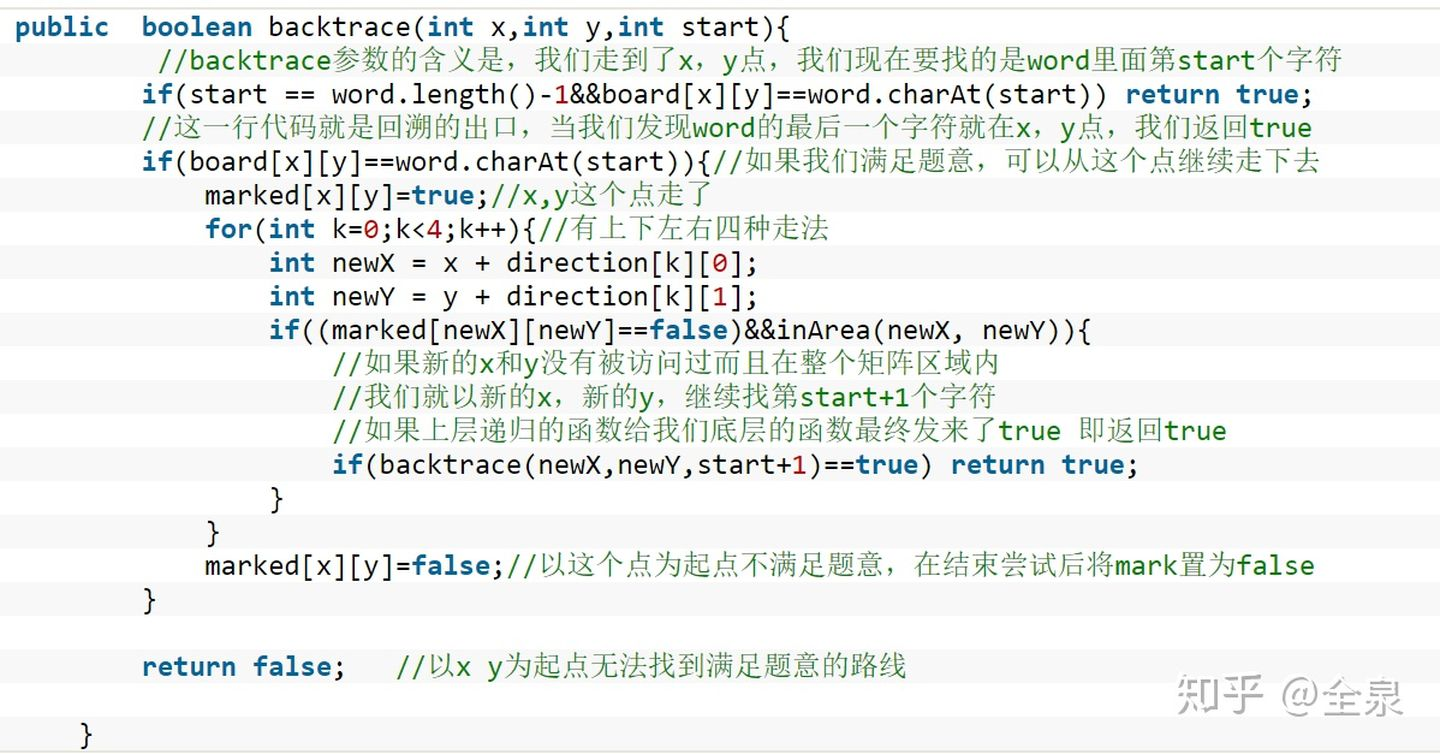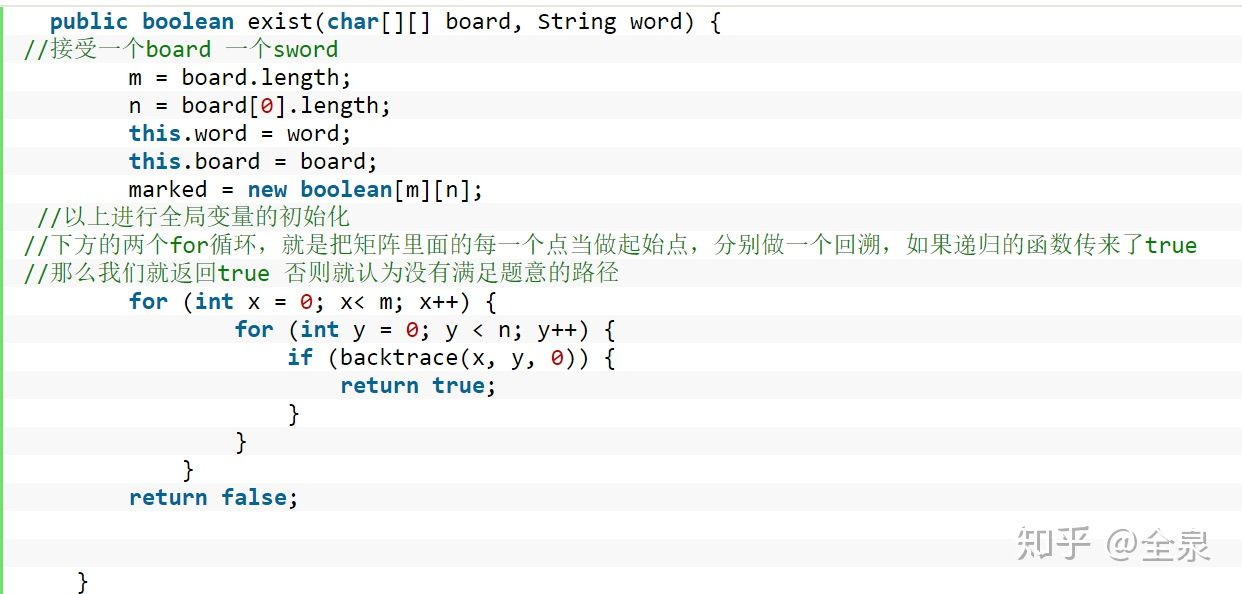﻿• 价格透明
• 信息保密
• 进度掌控
• 售后无忧告诉我，[回溯法]究竟是什么？浅谈回溯法的算法理解。。。

。。。

。。。

|D|        | |
|C|  回溯  |C|
|B|   -->  |B|
|A|        |A|
- -        - -

private boolean[][] marked; //记录这个点是不是被访问过了
private int[][] direction = {{-1, 0}, {0, -1}, {0, 1}, {1, 0}};//上下左右四个方向
private int m;// 盘面上有多少行
private int n;// 盘面上有多少列
private String word; //题目给出的word
private char[][] board; //题目给出的矩阵boardimport java.util.*;

class Solution {
private boolean[][] marked;
private int[][] direction = {{-1, 0}, {0, -1}, {0, 1}, {1, 0}};
// 盘面上有多少行
private int m;
// 盘面上有多少列
private int n;
private String word;
private char[][] board;

public  boolean backtrace(int x,int y,int start){
if(start == word.length()-1&&board[x][y]==word.charAt(start)) return true;
if(board[x][y]==word.charAt(start)){
marked[x][y]=true;//x,y这个点走了
for(int k=0;k<4;k++){//有上下左右四种走法
int newX = x + direction[k];
int newY = y + direction[k];
if((marked[newX][newY]==false)&&inArea(newX, newY)){

if(backtrace(newX,newY,start+1)==true) return true;
}
}
marked[x][y]=false;//以这个点为起点不满足题意，在结束尝试后将mark置为false
}

return false;   //以x y为起点无法找到满足题意的路线

}

public  boolean inArea(int x, int y) { //判断是否越界
return x >= 0 && x < m && y >= 0 && y < n;
}
public boolean exist(char[][] board, String word) {
m = board.length;
n = board.length;
this.word = word;
this.board = board;
marked = new boolean[m][n];
for (int x = 0; x< m; x++) {
for (int y = 0; y < n; y++) {
if (backtrace(x, y, 0)) {
return true;
}
}
}
return false;

}
}低价透明金牌服务信息保密